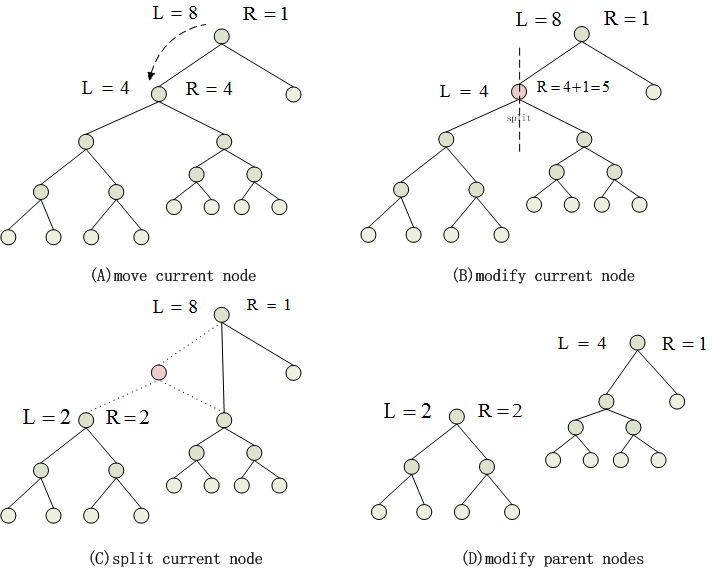## Fast clustering preprocessing• The step1 is executed on single machine , creating the init classes for the remaining data.
• The step2 is executed on the hadoop , all the data running in parallel .
• In order to make the most use of the Hadoop , the clusters number should maintain a certain proportion with the reduce number . According to the machine number of hadoop , we will set the reduce number manually. Assumeing the reduce number is k and the clusters number is h , the h will be limited to a certain proportion according to the k . For example , if h > 3k , we should merge two clusters with minimum nodes number. if h < k , we should split the clusters which has max number of nodes.

## Merge and Split

• All the clusters stored in tree structure. Every node in the tree saves two values ,L is the nodes number on the left sub tree and R is the nodes number of the right sub tree. The merge is very easy , just merge the root nodes of two sub trees.
• The split will be a little more complicated , as shown in the following figure.• The loading balancing process should make each cluster in the roughly same size , so after spliting, the ratio of two clusters should range in 1/2 to 2.
• (A)The initial node is at root , if L/R not in the 1/2 to 2 , move the current node to the sub nodes.
• (B)According to the parent node's information , modify the current node's L and R.
• (C)If current node satisfy the splitting condition, modify the pointer of the parent node and the sub nodes.
• (D)Along the motion path of current node to the root node, modify all the nodes' L and R values.

All Rights Reserved Copyright @ 2015|Dr. Quan Zou
Last Modified in 2016/7/26